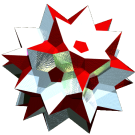Acronym pecu, J5 Name pentagonal cupola `© ©` Circumradius sqrt[sqrt(5)+11/4] = 2.232951 Vertex figures [3,4,5,4], [3,4,10] General of army (is itself convex) Colonel of regiment (is itself locally convex) Dihedral angles between {3} and {4}:   arccos(-(1+sqrt(5))/sqrt(12)) = 159.094843° between {4} and {5}:   arccos(-sqrt[(5+sqrt(5))/10]) = 148.282526° between {3} and {10}:   arccos(sqrt[(5+2 sqrt(5))/15]) = 37.377368° between {4} and {10}:   arccos(sqrt[(5+sqrt(5))/10]) = 31.717474° Confer general cupolae: n/d-cu   uniform relative: srid   related Johnson solids: pobcu   epcu   epobcu   pocuro   dirid   non-convex relative: rastacu   general polytopal classes: Johnson solids   segmentohedra ExternallinksAs abstract polytope pecu is isomorphic to stacu, thereby replacing the pentagon by a pentagram and the decagon by a Grünbaumian {10/2}. Or it is isomorphic to rastacu, thereby replacing the (prograde) pentagon by a retrograde pentagram and the decagon by a decagram.

This polyhedron is an edge-faceting of the small rhombicosidodecahedron (srid).

Incidence matrix according to Dynkin symbol

```xx5ox&#x   → height = sqrt((5-sqrt(5))/10) = 0.525731
({5} || {10})

o.5o.    | 5  * | 2  2 0 0 | 1 2 1 0
.o5.o    | * 10 | 0  1 1 1 | 0 1 1 1
---------+------+----------+--------
x. ..    | 2  0 | 5  * * * | 1 1 0 0
oo5oo&#x | 1  1 | * 10 * * | 0 1 1 0
.x ..    | 0  2 | *  * 5 * | 0 1 0 1
.. .x    | 0  2 | *  * * 5 | 0 0 1 1
---------+------+----------+--------
x.5o.    | 5  0 | 5  0 0 0 | 1 * * *
xx ..&#x | 2  2 | 1  2 1 0 | * 5 * *
.. ox&#x | 1  2 | 0  2 0 1 | * * 5 *
.x5.x    | 0 10 | 0  0 5 5 | * * * 1
```

```so10ox&#x   → height = sqrt((5-sqrt(5))/10) = 0.525731
({5} || {10})

demi( o.10o.    ) | 5  * | 2  2 0 0 | 1 1 0 2
.o10.o      | * 10 | 0  1 1 1 | 0 1 1 1
------------------+------+----------+--------
sefa( s.10o.    ) | 2  0 | 5  * * * | 1 0 0 1
demi( oo10oo&#x ) | 1  1 | * 10 * * | 0 1 0 1
demi( ..  .x    ) | 0  2 | *  * 5 * | 0 0 1 1
demi( ..  .x    ) | 0  2 | *  * * 5 | 0 1 1 0
------------------+------+----------+--------
s.10o.      | 5  0 | 5  0 0 0 | 1 * * *
demi( ..  ox&#x ) | 1  2 | 0  2 0 1 | * 5 * *
.o10.x      | 0 10 | 0  0 5 5 | * * 1 *
sefa( so10ox&#x ) | 2  2 | 1  2 1 0 | * * * 5

starting figure: xo10ox&#x
```